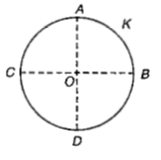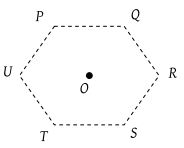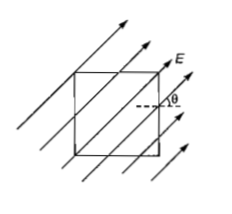Two parallel metal plates having charges +Q and -Q faces each other at a certain distance between them. If the plates are now dipped in kerosene oil tank, the electric field between the plates will

(a) become zero                                    (b) increase

(c) decrease                                          (d) remain same

Concept Questions :-

Electric field
High Yielding Test Series + Question Bank - NEET 2020

Difficulty Level:

The electric field at a distance $\frac{3\mathrm{R}}{2}$ from the centre of a charged conducting spherical shell of radius R is E. The electric field at a distance $\frac{\mathrm{R}}{2}$ from the centre of the sphere is

1. $\mathrm{zero}$                                     2. $E$

3. $\frac{E}{2}$                                       4. $\frac{E}{3}$

Concept Questions :-

Electric field
High Yielding Test Series + Question Bank - NEET 2020

Difficulty Level:

The mean free path of electrons in a metal is $4×{10}^{-8}m.$The electric field which can give on an average 2 eV energy to an electron in the metal will be in a unit of $V{m}^{-1}$ :

(a) $8×{10}^{7}$                              (b) $5×{10}^{-11}$

(c) $8×{10}^{-11}$                          (d) $5×{10}^{7}$

Concept Questions :-

Electric field
High Yielding Test Series + Question Bank - NEET 2020

Difficulty Level:

A thin conducting ring of radius R is given a charge +Q. The electric field at the centre O of the ring due to the charge on the part AKB of the ring is E. The electric field at the centre due to the charge on the part ACDB of the ring is(a) 3E along KO

(b) E along OK

(c) E along KO

(d) 3E along OK

Concept Questions :-

Electric field
High Yielding Test Series + Question Bank - NEET 2020

Difficulty Level:

There is a uniform electric field of strength 103 V/m along the y-axis. A body of mass 1g and charge 10–6C is projected into the field from the origin along the positive x-axis with a velocity 10m/s. Its speed in m/s after 10s is (Neglect gravitation)

(1) 10

(2) $5\sqrt{2}$

(3) $10\sqrt{2}$

(4) 20

Concept Questions :-

Electric field
High Yielding Test Series + Question Bank - NEET 2020

Difficulty Level:

Six charges, three positive and three negative of equal magnitude are to be placed at the vertices of a regular hexagon such that the electric field at O is double the electric field when only one positive charge of same magnitude is placed at R. Which of the following arrangements of charges is possible for P, Q, R, S, T and U respectively(1) +, –, +, –, –, +

(2) +, –, +, –, +, –

(3) +, +, –, +, –, –

(4) –, +, +, –, +, –

Concept Questions :-

Electric field
High Yielding Test Series + Question Bank - NEET 2020

Difficulty Level:

A surface of side L metre in the plane of the paper is placed in a uniform electric field E(volt/m) acting along the same place at an angle $\theta$ with the horizontal side of the square as shown in figure. The electric flux linked to the surface in unit of V-m, is(a) EL2                (b) EL2cos$\theta$

(c) EL2sin$\theta$          (d) 0

Concept Questions :-

Gauss law
High Yielding Test Series + Question Bank - NEET 2020

Difficulty Level:

Two positive ions, each carrying a charge q, are separated by a distance d. If F is the force of repulsion between the ions, the number of electrons missing from each ion will be (e being the charge on an electron)

1.                   2. $\sqrt{\frac{4{\mathrm{\pi \epsilon }}_{\circ }{\mathrm{Fe}}^{2}}{{d}^{2}}}$

3. $\sqrt{\frac{4{\mathrm{\pi \epsilon }}_{\circ }{\mathrm{Fd}}^{2}}{{e}^{2}}}$               4. $\frac{4{\mathrm{\pi \epsilon }}_{\circ }{\mathrm{Fd}}^{2}}{{q}^{2}}$

Concept Questions :-

Coulomb's law
High Yielding Test Series + Question Bank - NEET 2020

Difficulty Level:

A charge Q is enclosed by a Gaussian spherical surface of radius R. If the radius is doubled, then the outward electric flux will

1. be reduced to half

2. remain the same

3. be doubled

4. increase four times

Concept Questions :-

Gauss law
High Yielding Test Series + Question Bank - NEET 2020

Difficulty Level:

What is the flux through a cube of side a if a point charge of q is a one of its corner?

1. $\frac{2q}{{\epsilon }_{0}}$

2. $\frac{q}{8{\epsilon }_{0}}$

3. $\frac{q}{{\epsilon }_{0}}$

4. $\frac{q}{2{\epsilon }_{0}}6{a}^{2}$

Concept Questions :-

Gauss law